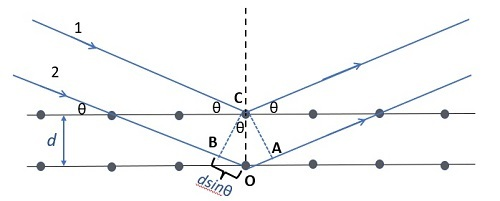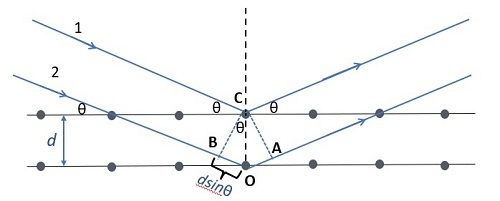# Bragg's Law - Definition, Derivation, Equation, Applications

## Introduction

Bragg’s law is an important concept of atomic and molecular physics. You are already aware that atoms are the building blocks of this universe. They are the foundation of every bit of matter in this universe. But that is not all that we know about the microscopic world. Indeed, we have even managed to divide the atom into electrons, protons, and neutrons. If that is not enough to satisfy your curiosity, you should know that we even know about what “makes up” the electrons and nucleons. But I digress.

For now, let us discuss how atoms are arranged. Inside a material, atoms or molecules reside at specific locations and most commonly, have some sort of periodicity within their arrangement. Further, different materials have different arrangements of atoms.

To study the placement of these atoms, we model the crystal as a set of discrete parallel planes on which the atoms reside. These planes are supposed to be equidistant. Modeling the crystal in terms of parallel planes allows us to carry out X-ray diffraction studies on the crystal. These studies are based on Bragg’s law. Let us discuss it in detail.

## Bragg’s Law?

In the figure given below, look at the two parallel planes, separated by a distance d, on which, X-ray radiation of wavelength λ is incident at an angle of θ.Bragg’s law states that the diffraction pattern observed by the waves reflected from the parallel planes in a crystal will have a maximum when the following condition is satisfied −

$$\mathrm{2d\:sin\:θ =n\lambda}$$

This is a very simple, yet highly powerful equation, which forms the foundation of a vast number of crystallographic studies. Note that since the interplanar spacing (d) is of the order of a few Angstroms, the most suitable wavelength for crystallographic studies lies in the X-ray region.

## Bragg’s Equation

Bragg equation refers to the mathematical statement of Bragg’s law. In its most common form, it is written as follows −

$$\mathrm{2d\:sin\:θ =n\lambda}$$

Here, d is the interplanar spacing, θ is the angle of incidence, and λ is the wavelength of incident radiation. Finally, n is a positive integer and corresponds to the order of diffraction.

Another statement of Bragg’s law is written in terms of the reciprocal lattice vector G, and the incident wavevector k as follows −

$$\mathrm{2 k.G+G^2= 0}$$

The above two statements are identical, but the second is defined in the reciprocal lattice.

## Derivation of Bragg’s Law

Bragg’s law can be derived using very simple rules of optics. Take a look at the diagram below −In this diagram, we have dropped three perpendiculars as follows −

• OC is perpendicular to the two planes shown above.

• BC is perpendicular to the incident ray.

• CA is perpendicular to the reflected ray.

From geometry, we can show that $\mathrm{\angle BCO = \theta}$. Now, using trigonometric ratios −

$$\mathrm{sin θ =\frac{perpendicular}{hypotenuse}=\frac{BO}{CO}}$$

Since CO = d, we have,

$$\mathrm{dsin\:\theta =BO}$$

Further, ΔCBO and ΔCAO can be shown to be congruent since they share a common hypotenuse and have two sets of equal angles. Thus, BO = AO. The path difference between rays 1 and 2 is given by BO + AO. That is, path difference, $\mathrm{\delta}$ is,

$$\mathrm{\delta=2BO=2d\:sin\:\theta}$$

For a maximum to occur, the path difference must be an integral multiple of the wavelength. Therefore, we arrive at the Bragg’s condition as follows −

$$\mathrm{2d\:sin\:\theta =n\lambda}$$

## Applications of Bragg’s Law

Bragg’s law has enormous applications in the fields of crystallography. Take a look −

• X-ray diffraction studies like Laue’s method, powdered crystal method, and rotating crystal method are based on Bragg’s law.

• X-ray fluorescence spectroscopy is based on the concepts of Bragg’s law.

## Importance of Bragg’s Law

The term Bragg’s diffraction is used to refer to the phenomenon of diffraction observed when electromagnetic radiation (basically light) is reflected from crystallographic planes. The diffraction occurs only when the wavelength of the radiation is comparable to the interplanar spacing, which occurs in the X-ray region.

Bragg’s diffraction is observed for a very specific set of parameters. For carrying out diffraction studies, we need to maximize the possibility of those parameters being satisfied. This is achieved by employing certain methods like Laue’s method, rotating crystal method, etc.

## Examples

Q1: If the interplanar spacing is 2.014 Angstrom, calculate the angle of the second-order diffraction, given wavelength is 1.544 Angstrom.

Ans: Bragg’s law gives $\mathrm{2d\:sin\:sin \:\theta =n\lambda}$

Solving for theta

$$\mathrm{\theta=\frac{n\lambda}{2d}=\frac{2\times 1.544\times 10^{−10}}{2\times2.014\times 10^{−10}}=0.766666 =50.05^{\circ}}$$

Q2: Given a crystal with an interplanar spacing of 3.03 Angstrom, find the angle of incidence for the first diffraction maximum. The energy of the incident neutron beam in this scenario is 0.1 eV. $\mathrm{(Mass\:of\:neutron = 1.67\times\:10^{−27}\:kg)}$.

Ans: The first step is to calculate the wavelength from the energy given. We use de Broglie’s formula.

$$\mathrm{\lambda=\frac{h}{\sqrt{2mqE}}=\frac{6.626\times 10^{−34}}{\sqrt{2×1.67\times 10^{−27}\times 1.6\times10^{−19}\times0.1}}=9.06\times 10^{−11}m}$$

Next, we use Bragg’s law to solve for θ

$$\mathrm{2d\:sin\:sin\:\theta =n\lambda}$$

$$\mathrm{\theta=\frac{n\lambda}{2d}=\frac{9.06\times 10^{−11}}{2\times 3.03\times 10^{−10}}=0.1495 =8.59^{\circ}}$$

Thus, the incident angle must be $\mathrm{8.59^{\circ}}$

## Conclusion

X-ray diffraction is an important technique for the characterization of crystals of solids. These diffraction studies are based on Bragg’s law, which models the crystal as a set of discrete parallel planes and then derives a relation between the interplanar spacing, the angle of incidence, and the wavelength of the incident radiation.

Let us suppose that the interplanar spacing is represented by d, the angle of incidence by θ, and the wavelength of incident radiation by λ. Then Bragg’s law states that the maximum of diffraction will be observed only when the following condition is satisfied −

$$\mathrm{2d\:sin\:\theta =n\lambda}$$

The derivation for Bragg’s law involves simple laws of geometrical optics. Bragg’s diffraction, which refers to diffraction observed due to the reflection of electromagnetic radiation by crystal planes, is used in X-ray diffraction of crystals and X-ray fluorescence spectroscopy.

## FAQs

Q1. Do all crystals allow diffraction for all crystal planes?

Ans. No. Different types of crystals have different ways in which the atoms are arranged. Thus, planes that allow diffraction in one type of crystal may not do so in other crystals. For instance, face-centered crystals show diffraction only when the Miller indices of the planes involved are either all odd or all even.

Q2. What kind of scattering is observed in Bragg’s law?

Ans. Rayleigh scattering is observed here since no change in wavelength occurs.

Q3. Can a single crystal have multiple values of interplanar spacing?

Ans. Yes. There are different sets of parallel planes in a crystal. These are characterized by their Miller indices, h,k, and l. The value of interplanar spacing depends on the Miller indices.

Q4. What does a typical X-ray diffraction spectrum look like?

Ans. The X-ray diffraction spectrum is plotted on a graph that shows intensity as a function of 2θ. Typically, it shows several peaks at specific values of θ, with the remaining areas being almost flat and zero.

Q5. Why is the XRD spectrum plotted against 2θ instead of θ?

Ans. This has to do with how crystal diffraction methods are designed. The outcome of diffraction is observed via detectors that are placed around the crystal. Often, the diffracted beams lie on a cone of angle 2θ. Thus, the XRD spectrum is plotted against 2θ.

Q6. Why is X-ray used in crystal diffraction?

Ans. The interplanar spacing in most crystals is similar in order of magnitude to the wavelength of X-rays. If the wavelength differs significantly from the interplanar spacing, the light will pass through the crystal without being diffracted. Thus, X-rays are used.

Updated on: 06-Apr-2023

610 Views